# Combination Formula using Recursion in C

## Combination Formula using Recursion in C with Examples

In this article, I am going to discuss the Combination Formula using Recursion in C Language with Examples. Please read our previous article where we discussed Taylor Series using Horner’s Rule by recursion in C Language with Examples. Before jumping on to the coding part and Tracing tree, first, we have to understand the concept.

##### Understanding Combination Formula:

First, understand what is Combination formula or Selection formula is then we create a recursive definition and we will write a recursive function for that one, and then we will analyze that function. If the set of objects are given to us, then in how many ways we can select the sub-set of those objects. This formula is used for finding the number of ways we can select the subset.

For example, if alphabets are given to us:

A B C D E F G And I want to select only 3, then in how many ways we can select any 3 of them? i.e., ABC, ABD. Interchanging of the position will not consider as it is known as permutation but we want a combination.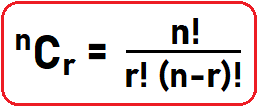Above is the formula to calculate nCr, let’s know more about it. In the above formula, r can be range from 0 to n i.e., 5C0-5 = 5C0, 5C1, 5C2, 5C3, 5C4, 5C5. So, let’s have a look at the code part.

##### Iterative Function:
```#include <stdio.h>
int Fact(int n)
{
int v = 1;
for (int i = 1; i <= n; i++)
{
v = v * i;
}
return v;
}

int NCR(int n, int r)
{
int x1, x2, x3;
x1 = Fact(n);
x2 = Fact(r);
x3 = Fact(n - r);

return x1 / (x2 * x3);
}

int main ()
{
int n, r;
n = 5;
r = 3;
printf ("%d", NCR(n, r));
}
```

Output: 10

Time Complexity: O(n)

This method uses the Fact function as we discuss factorial in our previous articles. Let’s look at its recursive version.

##### Recursive Function:
```#include <stdio.h>
int NCR (int n, int r)
{
if (r == 0 || n == r)
{
return 1;
}
else
return NCR (n - 1, r - 1) + NCR (n - 1, r);
}

int main ()
{
int n, r;
n = 5;
r = 3;
printf ("%d", NCR (n, r));
}
```

Output: 10

Time Complexity: O(n)

In the above function nCr, we take two-parameter n and r then we declare our base condition where function has to be terminated and return some basic value which we will use that value in other recursive calls. We will discuss it in diagram explanation or tracing tree part.
Here the base condition is:
If (r == 0 || n == r)
return 1;

This simply means when the value of r will 0 or equals to n then return 1 because we know the value of nC0 and nCr (where n = r) is 1.

else
return nCr (n – 1, r – 1) + nCr (n – 1, r);
In the else part, we return the addition of two recursive calls. To understand this, first, we have to understand Pascal’s Triangle. Below is the Pascal’s Triangle: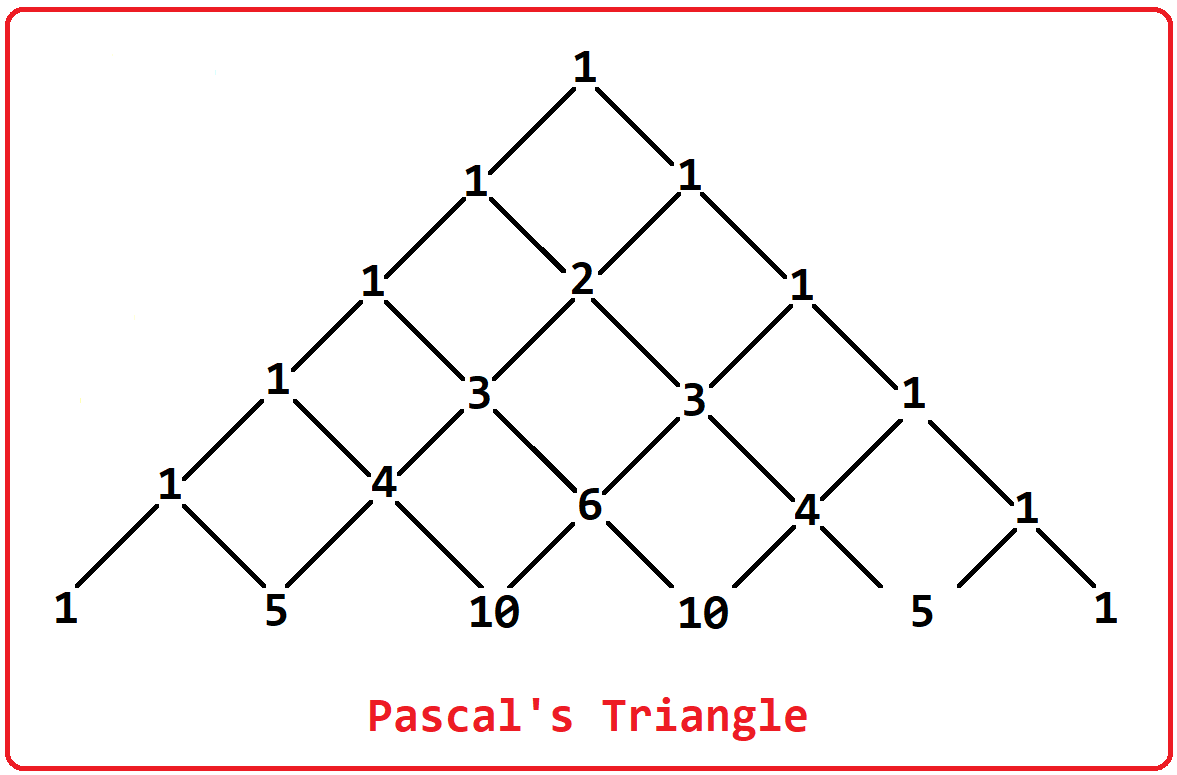In the above image, we can clearly see that the left and right side of the triangle contains only 1 and some numbers in the middle nodes. These numbers are not random here is a simple trick which is every next middle’s term is the sum of the previous two-term.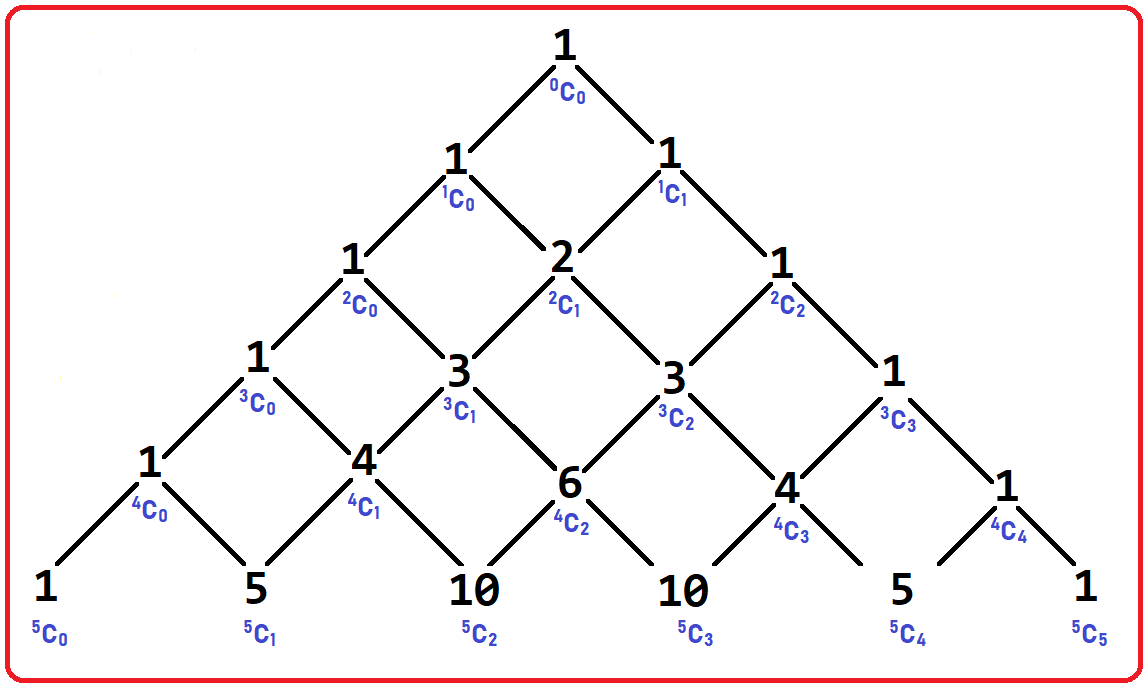We have modified the above Pascal’s Triangle as you can see the NCR values also. In the same manner, our recursive function will work. Let’s move to the Tracing tree part for more understanding.

#### Tracing Tree of Combination Formula using Recursion in C Language:

##### Step1: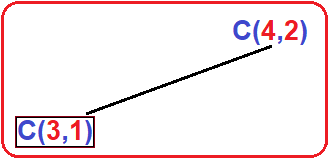Tree: we call our recursive function C (for here we call nCr function to only C) in our main program with n = 4 and r = 2. From here C (4,2) call will execute and inside this it checks for if (2 == 0 || 4 == 2)? No, then it goes to the else part which will return C (4-1, 2-1) + C (4-1, 2). Simply return the addition of C (3,1) and C (3, 2). But to know the result of addition, both the calls have to finish and return something then only we can calculate our result. Now to execute the second call i.e., C (3, 2), the first call has to finish i.e., C (3,1).

##### Step2: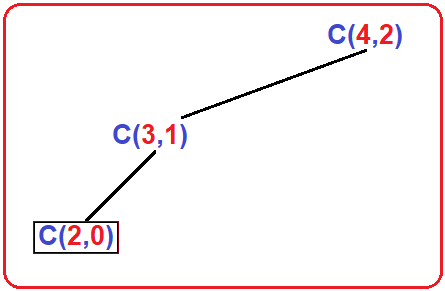Tree: Here, inside C (3,1) it will check for base condition if (1 == 0 || 3 == 1)? No, then it goes to else part and return C (3-1, 1-1) + C (3-1, 1) i.e., C (2, 0) + C (2, 1). But to know the result of addition, both the calls have to finish and return something then only we can calculate our result. Now to execute the second call i.e., C (2, 1), the first call has to finish i.e., C (2,0).

##### Step3: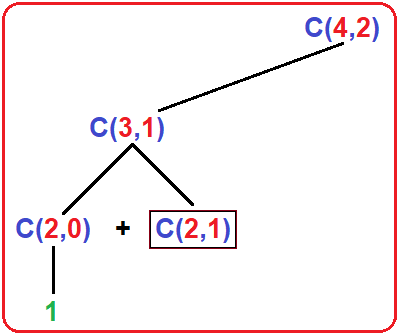Tree: In C (2, 0) call, it will check for if (0 == 0 || 2 == 0)? Yes, then here it will return 1 and it return to previous call i.e., C (3, 1) and from here it will execute it second call which is C (2, 1).

##### Step4: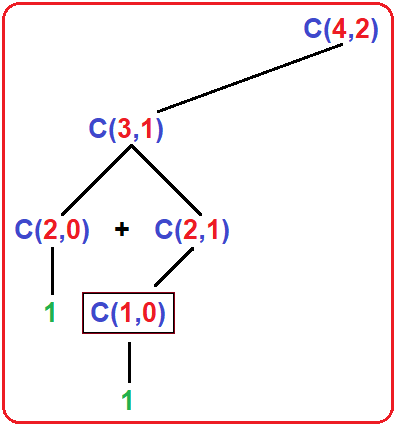Tree: In C (2, 1), it will check for if (1 == 0 || 2 == 1) No, then it goes to else part and return C (1, 0) + C (1, 1). In C (1, 0), it will check if (0 == 0 || 1 == 0) Yes, then it will return 1 to previous call i.e., C (2, 1) and from here it will execute it second call which is C (1, 1).

##### Step5: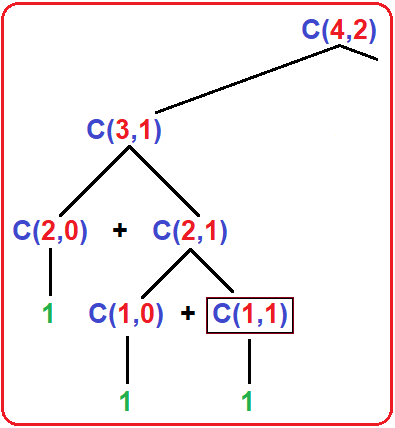Tree: In C (1, 1), it will check for if (1 == 0 || 1 == 1) Yes, then it will return 1 to the previous call i.e., C (2, 1) and now all calls of C (2, 1) have finished then control goes back to the previous call which is C (3, 1). Here also all calls have finished then control shift to C (4, 2).

##### Step6: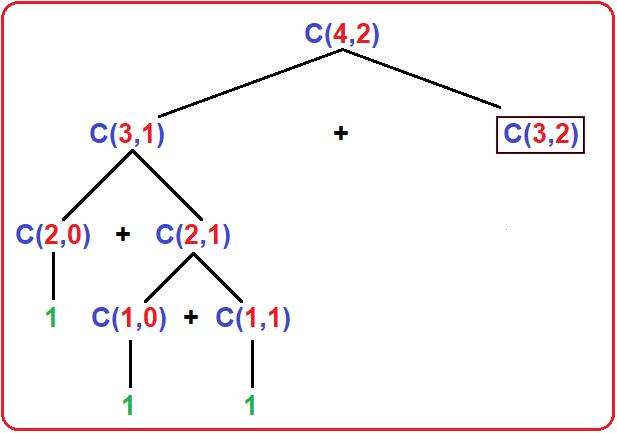Tree: Here C (4, 2) will make its 2nd call which is C (3, 2) as it finished its 1st call which is C (3, 1).

##### Step7: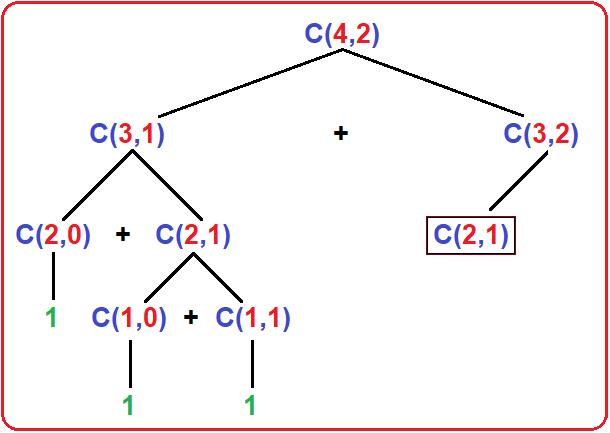Tree: In C (3, 2), it will check for if (2 == 0 || 3 == 2) No, then it goes to else part and return C (2, 1) + C (2, 2).

##### Step8: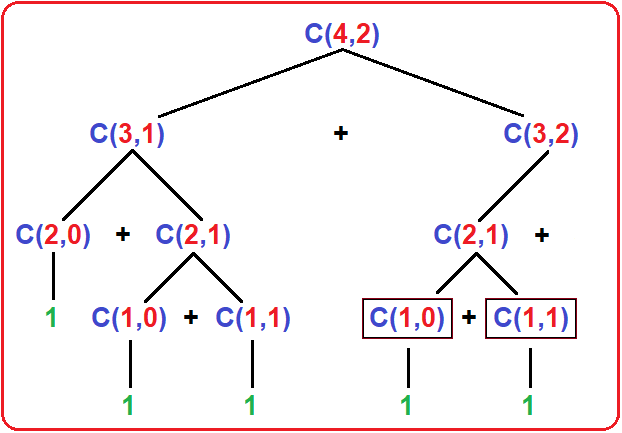Tree: In C (2, 1), it will check for if (1 == 0 || 2 == 1) No, then it goes to else part and return C (1, 0) + C (1, 1). In C (1, 0), it will check if (0 == 0 || 1 == 0) Yes, then it will return 1 to previous call i.e., C (2, 1) and from here it will execute it second call which is C (1, 1).

In C (1, 1), it will check for if (1 == 0 || 1 == 1) Yes, then it will return 1 to the previous call i.e., C (2, 1) and now all calls of C (2, 1) have finished then control goes back to the previous call which is C (3, 2).

##### Step9: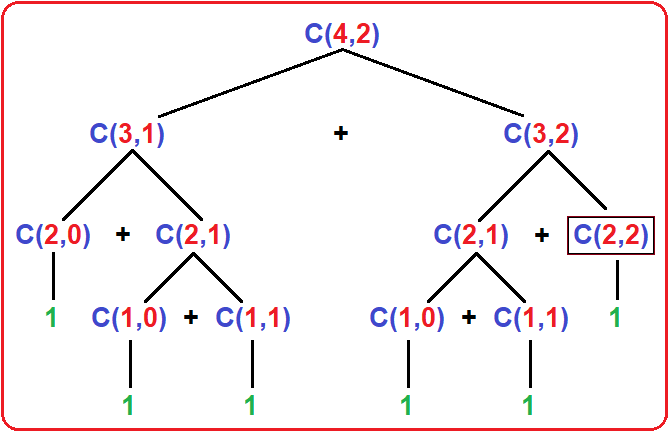Tree: Here C (3, 2) will make it 2nd call which is C (2, 2) and C (2, 2) will return 1 as the if condition satisfied. And here all calls of C (3, 2) as well as C (4, 2) have finished, and as we can see in the diagram where we use the ‘+’ sign which indicates the addition of the result of two calls. And finally, the result will print on the screen.

In the next article, I am going to discuss Fibonacci Series using Recursion in C Language with Examples. Here, in this article, I try to explain the Combination Formula or Selection Formula using Recursion in C Language with Examples and I hope you enjoy this Combination Formula using Recursion in C Language with Example article.